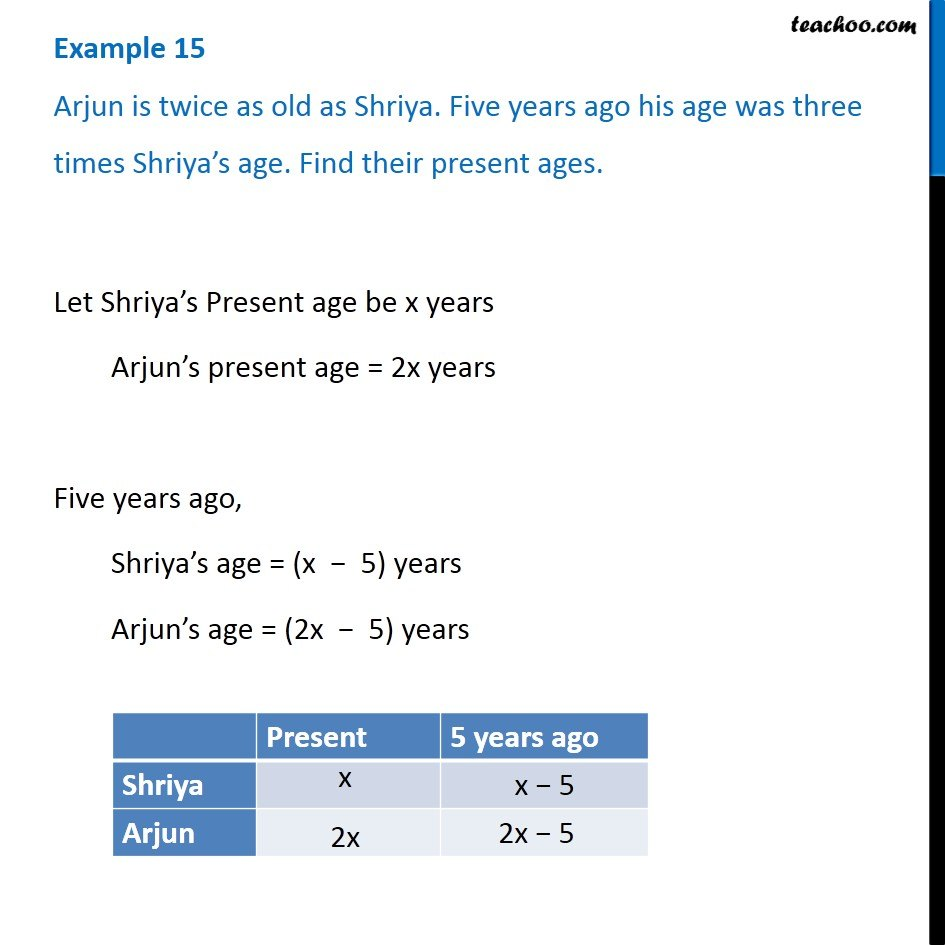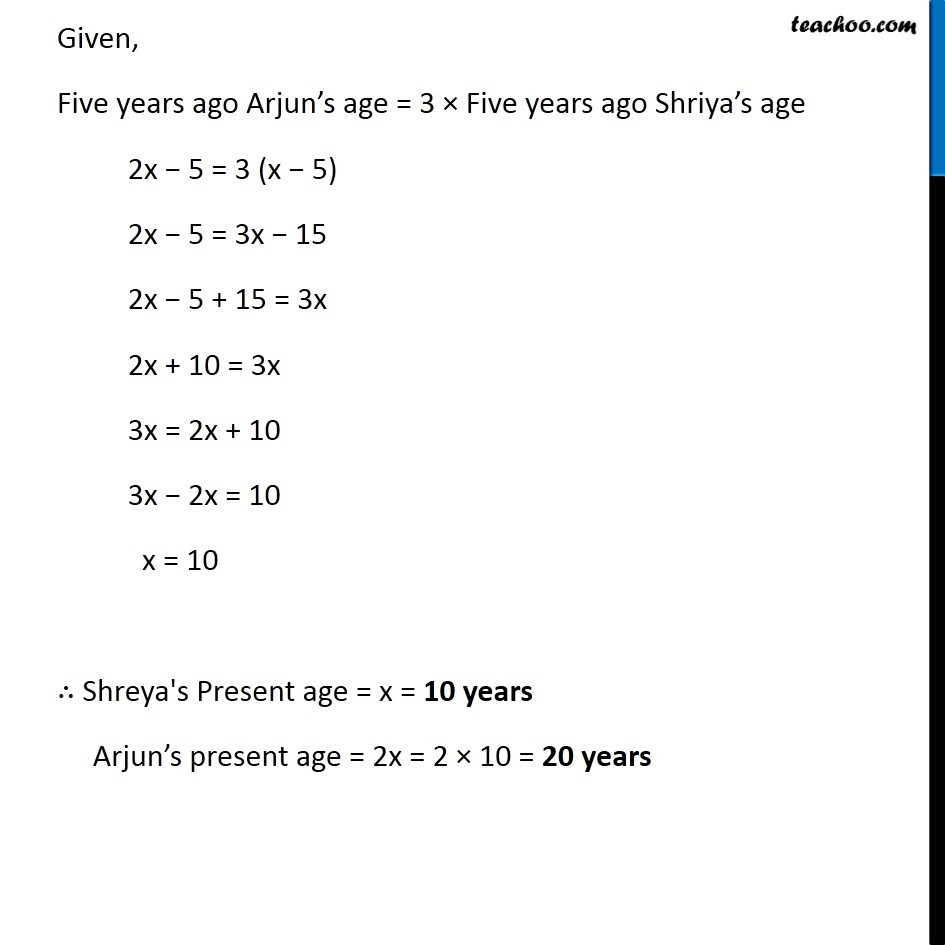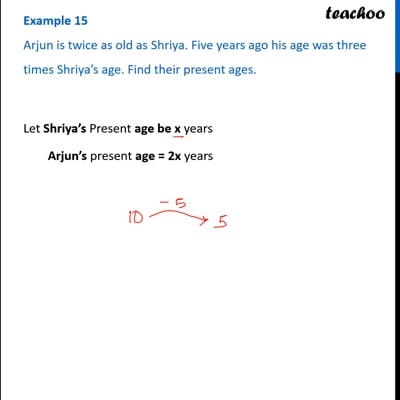Examples

Chapter 2 Class 8 Linear Equations in One Variable
Serial order wiseThis video is only available for Teachoo black users

Learn in your speed, with individual attention - Teachoo Maths 1-on-1 Class

### Transcript

Question 13 Arjun is twice as old as Shriya. Five years ago his age was three times Shriya s age. Find their present ages.Let Shriya s Present age be x years Arjun s present age = 2x years Five years ago, Shriya s age = (x 5) years Arjun s age = (2x 5) years Given, Five years ago Arjun s age = 3 Five years ago Shriya s age 2x 5 = 3 (x 5) 2x 5 = 3x 15 2x 5 + 15 = 3x 2x + 10 = 3x 3x = 2x + 10 3x 2x = 10 x = 10 Shreya's Present age = x = 10 years Arjun s present age = 2x = 2 10 = 20 years